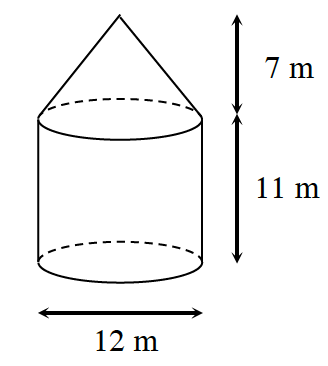### Home > INT2 > Chapter 11 > Lesson 11.2.3 > Problem11-85

11-85.A silo (a structure designed to store grain) has the shape of a cylinder with a cone on top, as shown in the diagram at right.

1. If a farmer wants to paint the silo, how much surface area must be painted?

Add the lateral areas of both shapes.

$LA_{\text{cone}} +LA_{\text{cylinder}}=\pi r\sqrt{h^2+r^2}+2\pi rh$

$=\pi (6)\sqrt{7^2+6^2}+2\pi(6)(11)$

$≈ 588.5\text{ m}^2$

2. What is the volume of the silo? That is, how many cubic meters of grain can the silo hold?

Just as you added the lateral areas of the two shapes to find the total surface area in part (a),
add the volumes of the two shapes to find the total volume.